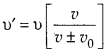Enlightened

# Question 15: NCERT Solutions for 12th Class Physics: Chapter 10-Wave Optics

• 0

Question 15: NCERT Solutions for 12th Class Physics: Chapter 10-Wave Optics

For sound waves, the Doppler formula for frequency shift differs slightly between the two situations :
(i) source at rest; observer moving, and
(ii) source moving; observer at rest.
The exact Doppler formulas for the case of light waves in vacuum are, however, strictly identical for these situations. Explain why this should be so. Would you expect the formulas to be strictly identical for the two situations in case of light travelling in a medium?

Share

1. Solution:
Sound waves require a medium for propagation. The Doppler formula for frequency shift differs slightly in two situations
(i) Source at rest, observer moving
(ii) Observer at rest, source movingThe two formulas are different because motion of the observer relative to the medium is different in the two situations for light waves in vacuum. No such relative relation of observer and medium exist. Hence only the relative motion between the source and the observer counts and theCheck the complete chapter with solutions.

NCERT Solutions for 12th Class Physics: Chapter 10-Wave Optics

• 0